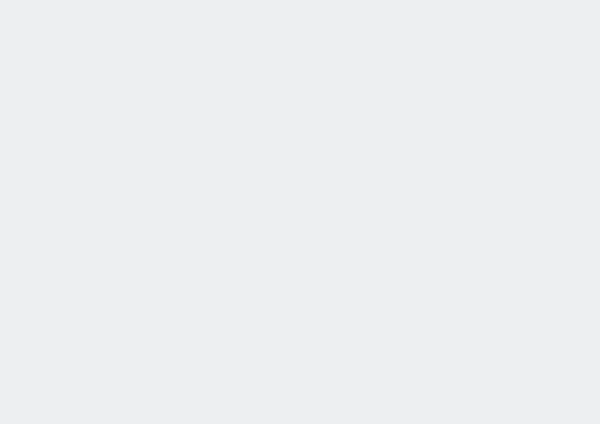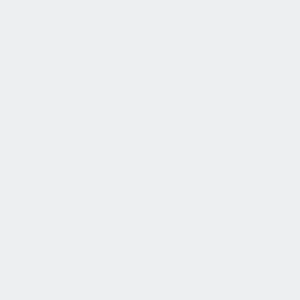Here’s Mathematician KP Hart’s Math Question and Answer for Monday, January 25th!

Aren’t there too many assumptions in science?

Science builds its theories from existing assumptions such as the number zero. How can the conclusions then be seen as true?

A similar question was discussed in this post. I do not know about All of Science but I can speak for Mathematics: we do our utmost to get rid of superfluous assumptions.

A classical example is Euclidean Geometry. The five assumptions that Euclid made (his postulates) were enough to able to derive all of the known geometry of that time. Many mathematicians thought his fifth assumption, the Parallel Postulate, somewhat special; it is not as short and succinct as the other four. It also almost sounds like a theorem. Through the centuries a lot of effort was spent to derive the fifth postulate from the other four. In the 19th century it became clear, and it was proven to be so, that the fifth postulate can not be derived from the other four. There are useful, non-Euclidean, geometries where the parallel postulate does not hold and that actually demonstrate the non-derivability of that postulate. This means that various theorems from Euclidean geometry, such as “the angles in a triangle add up to 180C degrees” do need the fifth postulate in their proofs.

Every part of mathematics has its axioms that describe the desired behavior of the objects of study, and about the first thing that happens when opening up such a new area is investigating whether there are assumptions that can be derived from the others. If yes, then they are put among the theorems, and if no, then they remain among the assumptions. Sometimes an assumption is used in special cases only and that tends to lead to mentioning it explicitly as an assumption in theorems. A good example is the Axiom of Choice in Set Theory.

The only problem of `too many assumptions’ can be their mutual consistency. But that is something where extra care is taken when building new theories: if the assumptions together imply something that is false then some of the assumptions must be scrapped.

As to the number zero: that has proved to be very useful.

*********************************

Read all of KP Harts math questions here!About Dutch Mathematician KP Hart: In the beginning of this year the Dutch government opened a website, The Dutch Science Agenda, where everyone could post questions that they thought were of scientific interest. This was an attempt to involve the whole country in determining what the Dutch science agenda should be in the coming years.

I looked through the questions and searched for terms like `mathematics’, `infinity’ … to see what mathematical questions there were and I noticed various questions that already have answers (and have had for a long time). On a whim I decided to post answers to those questions, in Dutch. For your edification I will translate these posts into English.